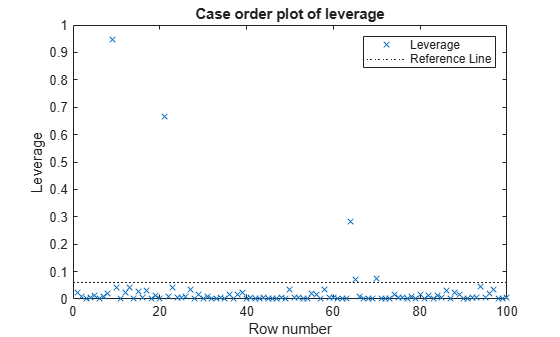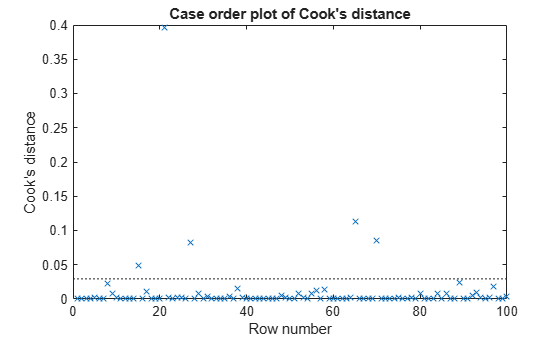# plotDiagnostics

Plot observation diagnostics of generalized linear regression model

## Syntax

``plotDiagnostics(mdl)``
``plotDiagnostics(mdl,plottype)``
``plotDiagnostics(mdl,plottype,Name,Value)``
``h = plotDiagnostics(___)``

## Description

`plotDiagnostics` creates a plot of observation diagnostics, such as leverage and Cook's distance, to identify outliers and influential observations.

example

````plotDiagnostics(mdl)` creates a leverage plot of the generalized linear regression model (`mdl`) observations. A dotted line in the plot represents the recommended threshold values.```

example

````plotDiagnostics(mdl,plottype)` specifies the type of observation diagnostics `plottype`.```
````plotDiagnostics(mdl,plottype,Name,Value)` specifies the graphical properties of diagnostic data points using one or more name-value pair arguments. For example, you can specify the marker symbol and size for the data points.```
````h = plotDiagnostics(___)` returns graphics objects for the lines or contour in the plot using any of the input argument combinations in the previous syntaxes. Use `h` to modify the properties of a specific line or contour after you create the plot. For a list of properties, see Line Properties and Contour Properties.```

## Examples

collapse all

Create leverage and Cook's distance plots of a fitted generalized linear model, and find the outliers.

Generate sample data using Poisson random numbers with two underlying predictors `X(:,1)` and `X(:,2)`.

```rng('default') % For reproducibility rndvars = randn(100,2); X = [2 + rndvars(:,1),rndvars(:,2)]; mu = exp(1 + X*[1;2]); y = poissrnd(mu);```

Create a generalized linear regression model of Poisson data.

`mdl = fitglm(X,y,'y ~ x1 + x2','Distribution','poisson');`

Create a leverage plot.

```plotDiagnostics(mdl) legend('show') % Show the legend```The dotted line represents the recommended threshold value 2*p/n, where p is the number of coefficients, and n is the number of observations. Find the threshold value using the `NumCoefficients` and `NumObservations` properties.

`t_leverage = 2*mdl.NumCoefficients/mdl.NumObservations`
```t_leverage = 0.0600 ```

Find the observations with leverage values that exceed the threshold value.

`find(mdl.Diagnostics.Leverage > t_leverage)`
```ans = 5×1 9 21 64 65 70 ```

You can also find an observation number by using a data tip. Select the data points above the threshold line to display their data tips. The data tip includes the x-axis and y-axis values for the selected point, along with the observation number.

Plot the Cook's distance values.

`plotDiagnostics(mdl,'cookd')`The dotted line represents the recommended threshold value. Compute the threshold value `t_cookd`.

`t_cookd = 3*mean(mdl.Diagnostics.CooksDistance')`
```t_cookd = 0.0294 ```

Find the observations with the Cook's distance values that exceed the threshold value.

`find(mdl.Diagnostics.CooksDistance > t_cookd)`
```ans = 5×1 15 21 27 65 70 ```

Three observations (21, 65, and 70) are outliers by both measures, but some points (9, 15, 27, and 64) are outliers by only one measure.

## Input Arguments

collapse all

Generalized linear regression model, specified as a `GeneralizedLinearModel` object created using `fitglm` or `stepwiseglm`.

Type of plot, specified as one of the values in this table.

ValuePlot TypeDotted Reference Line in Plot Purpose
`'contour'`Residual vs. leverage with overlaid contours of Cook's distanceContours of Cook's distanceIdentify observations with large residual values, high leverage, and large Cook's distance values.
`'cookd'`Cook's distanceRecommended threshold, computed by `3*mean(mdl.Diagnostics.CooksDistance)`Identify observations with large Cook's distance values.
`'leverage'`LeverageRecommended threshold, computed by `2*p/n`, where `p` is the number of coefficients (`mdl.NumCoefficients`) and `n` is the number of observations (`mdl.NumObservations`)Identify high leverage observations.

For `'cookd'` and `'leverage'`, the x-axis is the row number (case order) of observations.

The `Diagnostics` property of `mdl` contains the diagnostic values used by `plotDiagnostics` to create plots.

### Name-Value Arguments

Specify optional comma-separated pairs of `Name,Value` arguments. `Name` is the argument name and `Value` is the corresponding value. `Name` must appear inside quotes. You can specify several name and value pair arguments in any order as `Name1,Value1,...,NameN,ValueN`.

Example: `'Color','blue','Marker','o'`

Note

The graphical properties listed here are only a subset. For a complete list, see Line Properties. The specified properties determine the appearance of diagnostic data points.

Line color, specified as the comma-separated pair consisting of `'Color'` and an RGB triplet, hexadecimal color code, color name, or short name for one of the color options listed in the following table.

The `'Color'` name-value pair argument also determines marker outline color and marker fill color if `'MarkerEdgeColor'` is `'auto'` (default) and `'MarkerFaceColor'` is `'auto'`.

For a custom color, specify an RGB triplet or a hexadecimal color code.

• An RGB triplet is a three-element row vector whose elements specify the intensities of the red, green, and blue components of the color. The intensities must be in the range `[0,1]`; for example, ```[0.4 0.6 0.7]```.

• A hexadecimal color code is a character vector or a string scalar that starts with a hash symbol (`#`) followed by three or six hexadecimal digits, which can range from `0` to `F`. The values are not case sensitive. Thus, the color codes `'#FF8800'`, `'#ff8800'`, `'#F80'`, and `'#f80'` are equivalent.

Alternatively, you can specify some common colors by name. This table lists the named color options, the equivalent RGB triplets, and hexadecimal color codes.

Color NameShort NameRGB TripletHexadecimal Color CodeAppearance
`'red'``'r'``[1 0 0]``'#FF0000'``'green'``'g'``[0 1 0]``'#00FF00'``'blue'``'b'``[0 0 1]``'#0000FF'``'cyan'` `'c'``[0 1 1]``'#00FFFF'``'magenta'``'m'``[1 0 1]``'#FF00FF'``'yellow'``'y'``[1 1 0]``'#FFFF00'``'black'``'k'``[0 0 0]``'#000000'``'white'``'w'``[1 1 1]``'#FFFFFF'``'none'`Not applicableNot applicableNot applicableNo color

Here are the RGB triplets and hexadecimal color codes for the default colors MATLAB® uses in many types of plots.

`[0 0.4470 0.7410]``'#0072BD'``[0.8500 0.3250 0.0980]``'#D95319'``[0.9290 0.6940 0.1250]``'#EDB120'``[0.4940 0.1840 0.5560]``'#7E2F8E'``[0.4660 0.6740 0.1880]``'#77AC30'``[0.3010 0.7450 0.9330]``'#4DBEEE'``[0.6350 0.0780 0.1840]``'#A2142F'`Example: `'Color','blue'`

Line width, specified as the comma-separated pair consisting of `'LineWidth'` and a positive value in points. If the line has markers, then the line width also affects the marker edges.

Example: `'LineWidth',0.75`

Marker symbol, specified as the comma-separated pair consisting of `'Marker'` and one of the values in this table.

MarkerDescriptionResulting Marker
`'o'`Circle`'+'`Plus sign`'*'`Asterisk`'.'`Point`'x'`Cross`'_'`Horizontal line`'|'`Vertical line`'s'`Square`'d'`Diamond`'^'`Upward-pointing triangle`'v'`Downward-pointing triangle`'>'`Right-pointing triangle`'<'`Left-pointing triangle`'p'`Pentagram`'h'`Hexagram`'none'`No markersNot applicable

Example: `'Marker','+'`

Marker outline color, specified as the comma-separated pair consisting of `'MarkerEdgeColor'` and an RGB triplet, hexadecimal color code, color name, or short name for one of the color options listed in the `Color` name-value pair argument.

The default value of `'auto'` uses the same color specified by using `'Color'`.

Example: `'MarkerEdgeColor','blue'`

Marker fill color, specified as the comma-separated pair consisting of `'MarkerFaceColor'` and an RGB triplet, hexadecimal color code, color name, or short name for one of the color options listed in the `Color` name-value pair argument.

The `'auto'` value uses the same color specified by using `'Color'`.

Example: `'MarkerFaceColor','blue'`

Marker size, specified as the comma-separated pair consisting of `'MarkerSize'` and a positive value in points.

Example: `'MarkerSize',2`

## Output Arguments

collapse all

Graphics objects corresponding to the lines or contour in the plot, returned as a graphics array. Use dot notation to query and set properties of the graphics objects. For details, see Line Properties and Contour Properties.

You can use name-value pair arguments to specify the appearance of diagnostic data points corresponding to the first graphics object `h(1)`.

collapse all

### Cook’s Distance

Cook’s distance is the scaled change in fitted values, which is useful for identifying outliers in the observations for predictor variables. Cook’s distance shows the influence of each observation on the fitted response values. An observation with Cook’s distance larger than three times the mean Cook’s distance might be an outlier.

The Cook’s distance Di of observation i is

`${D}_{i}={w}_{i}\frac{{e}_{i}^{2}}{p\stackrel{^}{\phi }}\frac{{h}_{ii}}{{\left(1-{h}_{ii}\right)}^{2}},$`

where

• $\stackrel{^}{\phi }$ is the dispersion parameter (estimated or theoretical).

• ei is the linear predictor residual, $g\left({y}_{i}\right)-{x}_{i}\stackrel{^}{\beta }$, where

• g is the link function.

• yi is the observed response.

• xi is the observation.

• $\stackrel{^}{\beta }$ is the estimated coefficient vector.

• p is the number of coefficients in the regression model.

• hii is the ith diagonal element of the Hat Matrix H.

### Leverage

Leverage is a measure of the effect of a particular observation on the regression predictions due to the position of that observation in the space of the inputs.

The leverage of observation i is the value of the ith diagonal term hii of the hat matrix H. Because the sum of the leverage values is p (the number of coefficients in the regression model), an observation i can be considered an outlier if its leverage substantially exceeds p/n, where n is the number of observations.

### Hat Matrix

The hat matrix is a projection matrix that projects the vector of response observations onto the vector of predictions.

The hat matrix H is defined in terms of the data matrix X and a diagonal weight matrix W:

H = X(XTWX)–1XTWT.

W has diagonal elements wi:

`${w}_{i}=\frac{{g}^{\prime }\left({\mu }_{i}\right)}{\sqrt{V\left({\mu }_{i}\right)}},$`

where

• g is the link function mapping yi to xib.

• ${g}^{\prime }$ is the derivative of the link function g.

• V is the variance function.

• μi is the ith mean.

The diagonal elements Hii satisfy

`$\begin{array}{l}0\le {h}_{ii}\le 1\\ \sum _{i=1}^{n}{h}_{ii}=p,\end{array}$`

where n is the number of observations (rows of X), and p is the number of coefficients in the regression model.

## Tips

• The data cursor displays the values of the selected plot point in a data tip (small text box located next to the data point). The data tip includes the x-axis and y-axis values for the selected point, along with the observation name or number.

• Use `legend('show')` to show the pre-populated legend.

## Alternative Functionality

A `GeneralizedLinearModel` object provides multiple plotting functions.

 Neter, J., M. H. Kutner, C. J. Nachtsheim, and W. Wasserman. Applied Linear Statistical Models, Fourth Edition. Chicago: McGraw-Hill Irwin, 1996.